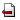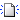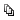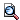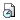The basic problem of the stability of functional equations asks whether an `approximate solution' of the so called Cauchy (or additive) functional equation f(x+y) = f(x)+f(y) `can be approximated' by a solution of this equation. This problem was formulated and solved in Gy. Pólya and G. Szeg″o's famous book Aufgaben und Lehrsätze aus der Analysis in the context of natural numbers in 1925. The question was (re)formulated in a more general setting by S. Ulam in 1940 and answered by D. H. Hyers in 1941. In this talk, we present several generalizations of Pólya and Szeg″o's and Hyers' results for a class of linear functional equations, the so called monomial equations.

ご不明な点がございましたら、事務局: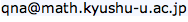までお問い合わせ下さい。